### Create an Account

Home / Questions / Equation 17 9 for the thermal shock resistance of a material is valid for relatively low r...

# Equation 17 9 for the thermal shock resistance of a material is valid for relatively low rates of heat transfer width When the rate is high then upon cooling of a body the maximum

Equation 17.9, for the thermal shock resistance of a material, is valid for relatively low rates of heat transfer.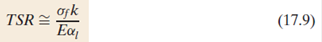When the rate is high, then, upon cooling of a body, the maximum temperature change allowable without thermal shock, ΔTf , is approximately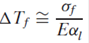where σf is the fracture strength. Using the data in Tables B.2, B.4, and B.6 (Appendix B)

Table B.2 Room-Temperature Modulus of Elasticity Values for Various Engineering Materials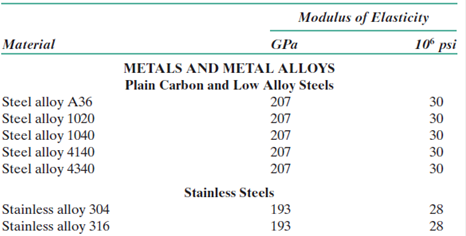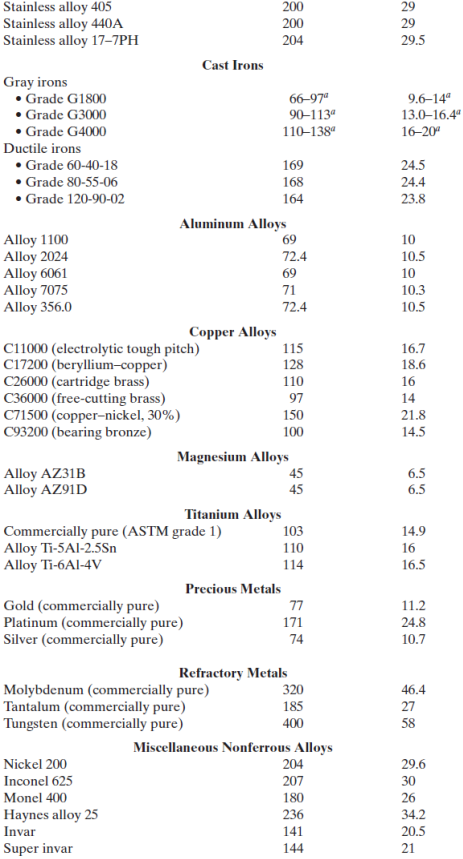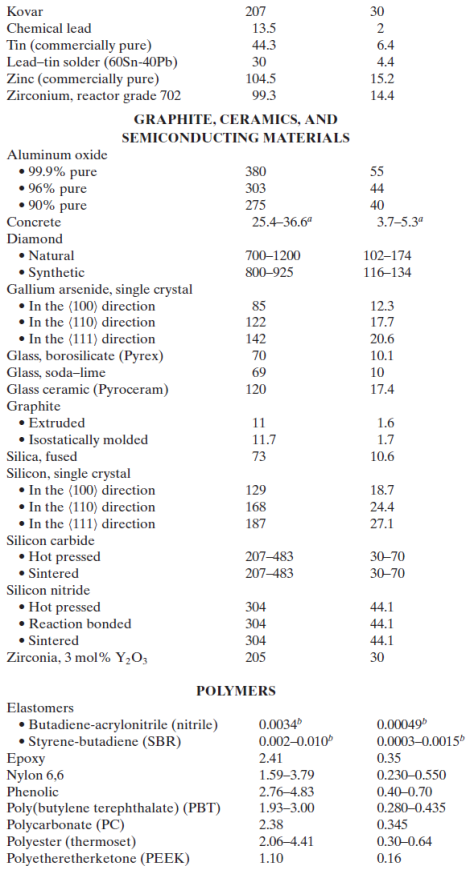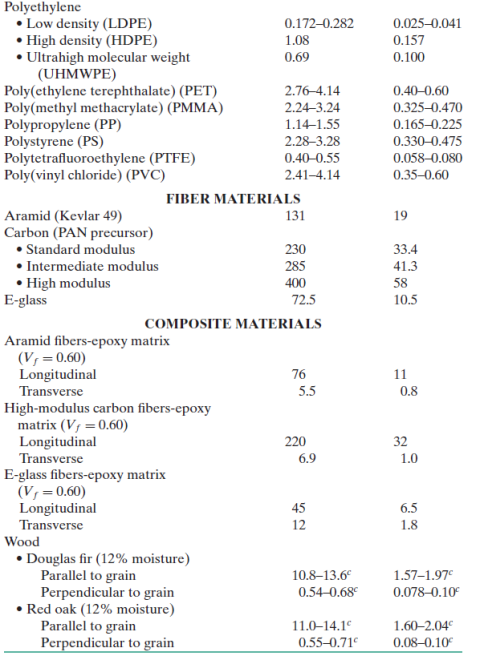Table B.4 Typical Room-Temperature Yield Strength, Tensile Strength, and Ductility (Percent Elongation) Values for Various Engineering Materials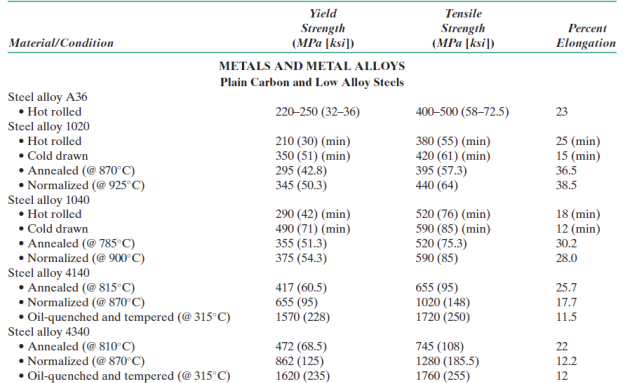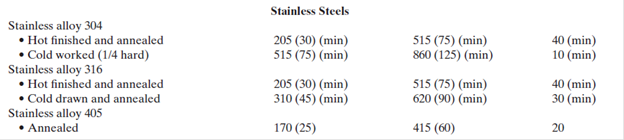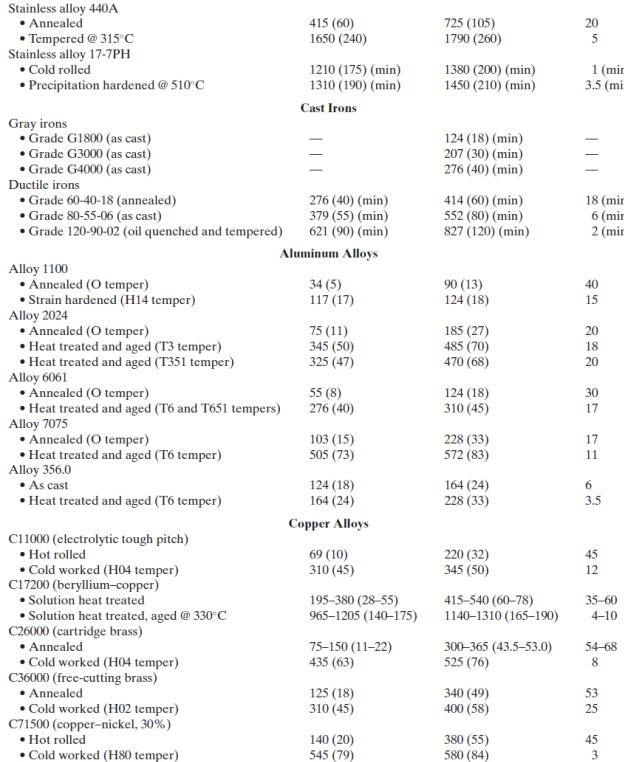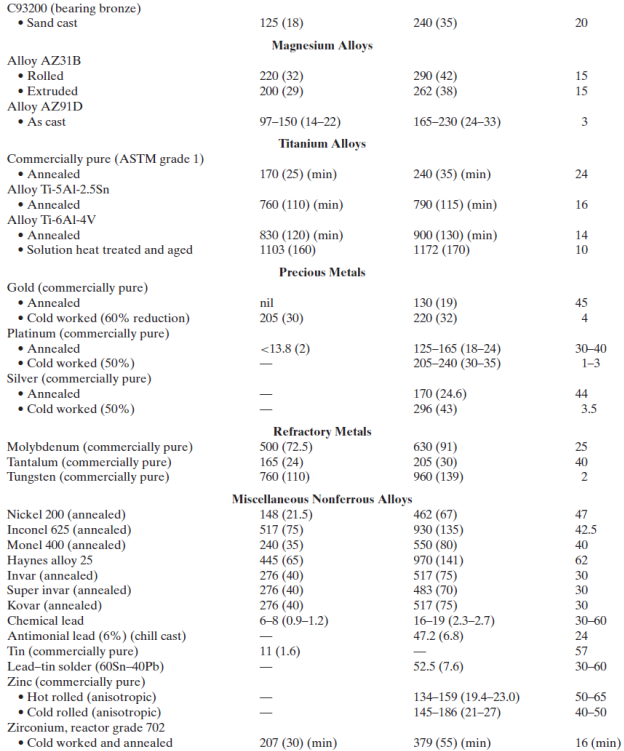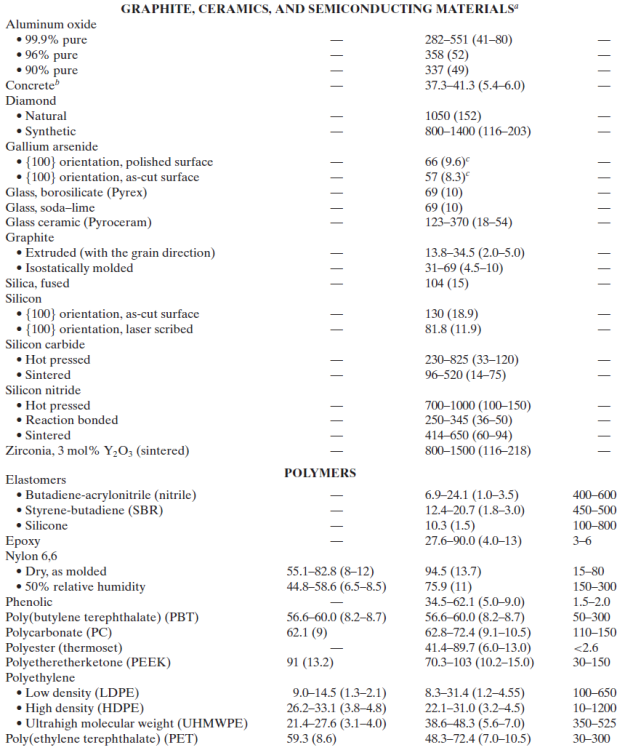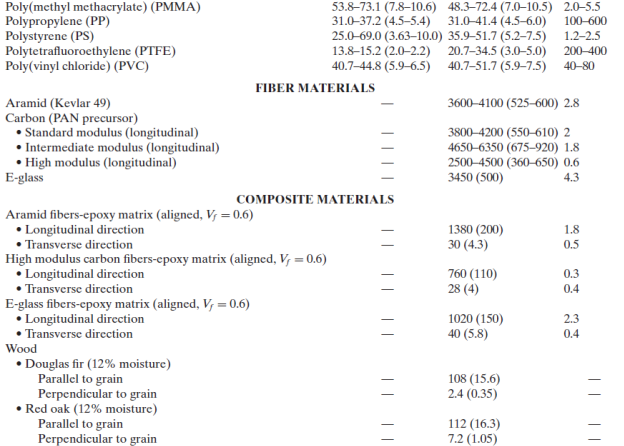Table B.6 Room-Temperature Linear Coefficient of Thermal Expansion Values for Various Engineering Materials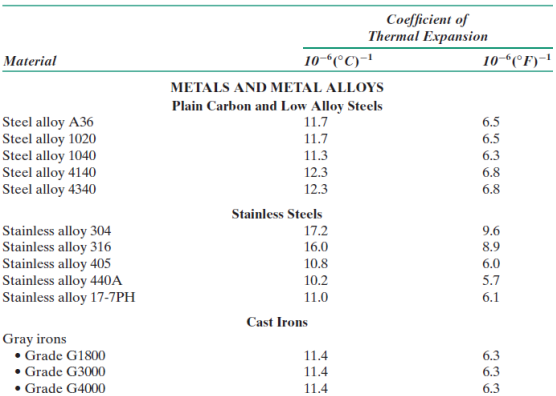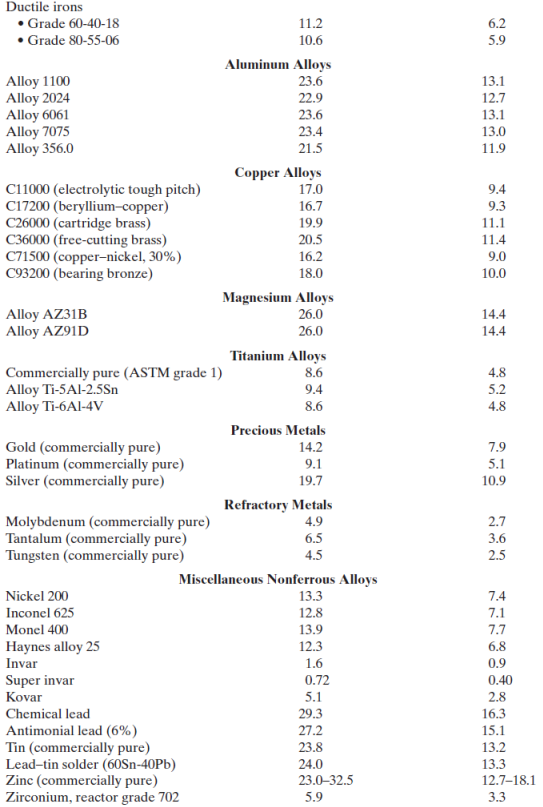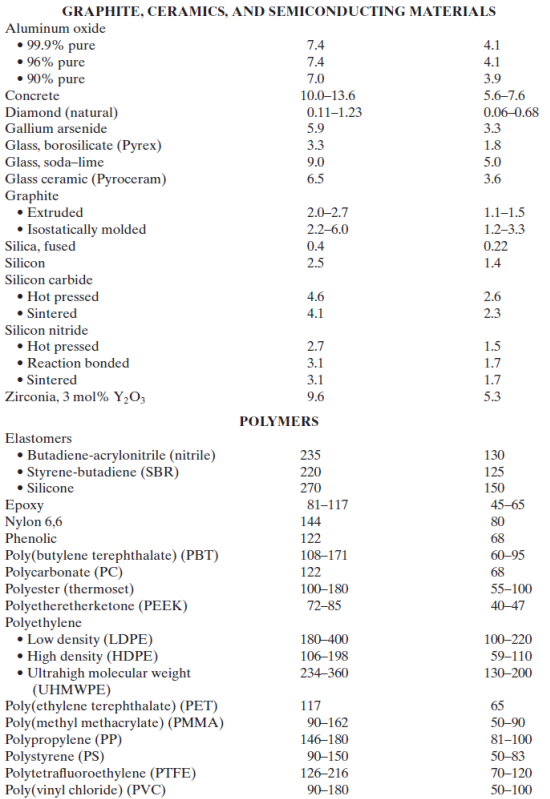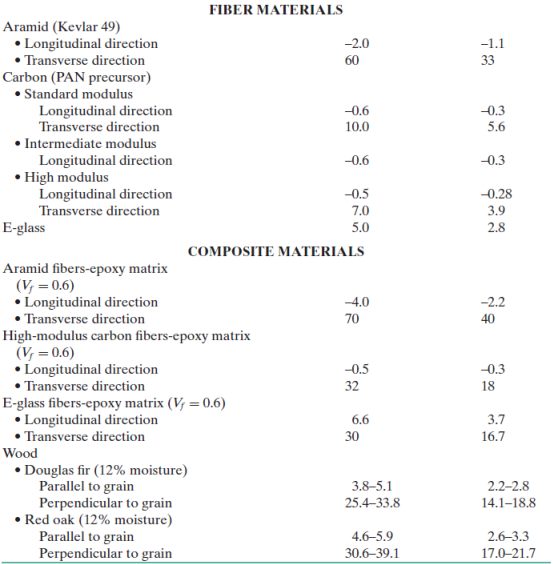Determine ΔTf for a soda–lime glass, borosilicate (Pyrex) glass, aluminum oxide (96% pure), and gallium arsenide [<100> direction and {100} orientation, as-cut surface].

May 26 2020 View more View LessSubscribe To Get Solution